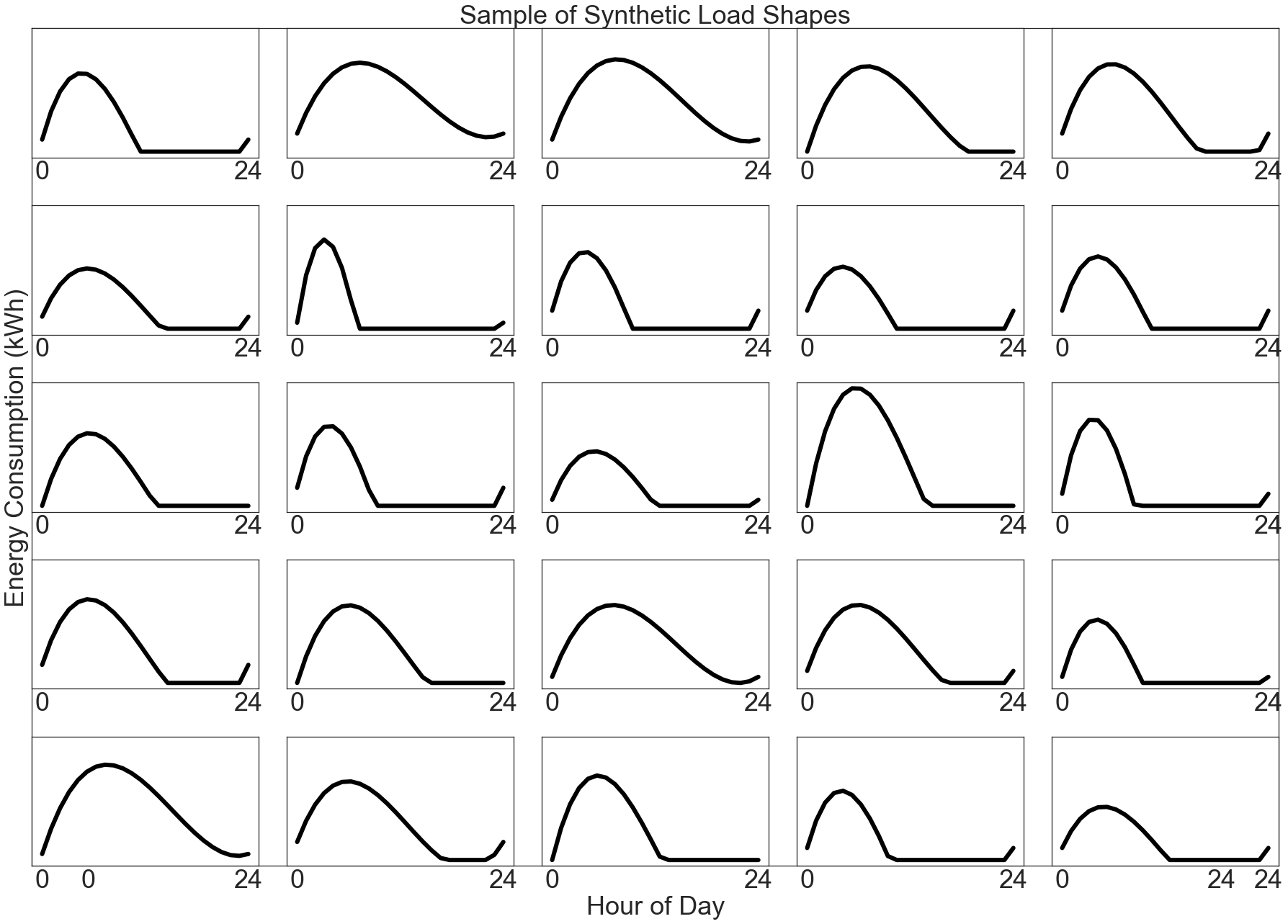# Private Comparison Groups¶

“Comparison Groups” is a powerful technique for isolating the effect of external influence on energy consumption. This technique has received growing attention because of the massive effect of COVID-19 quarantine measures on energy usage.

This notebook applies the methods described in Differentially Private Comparison Groups: Motivation and Methods, computing a number of useful Comparison Group statistics while preserving the privacy of individuals chosen for analysis.

:

# Preamble: imports and figure settings

from eeprivacy import (
PrivateVectorClampedMean,
)

import matplotlib.pyplot as plt
import numpy as np
import pandas as pd
import matplotlib as mpl
from scipy import stats

np.random.seed(1234) # Fix seed for deterministic documentation

mpl.style.use("seaborn-white")

MD = 28
LG = 36
plt.rcParams.update({
"figure.figsize": [25, 18],
"legend.fontsize": MD,
"axes.labelsize": LG,
"axes.titlesize": LG,
"xtick.labelsize": LG,
"ytick.labelsize": LG,
})


## Dataset¶

For this example, we generate a population of synthetic daily load shapes:

:

def synthetic_load_shape():
x = np.array([
0,
np.random.randint(2, 6),
np.random.randint(7, 18),
24
])
y0 = np.random.randint(low=0, high=4)
y = np.array([
y0,
np.random.randint(low=8, high=14),
np.random.randint(low=0, high=9),
y0
])
z = np.polyfit(x, y, 3)
p = np.poly1d(z)
xs = np.linspace(0, 24, num=24)
ys = p(xs)
ys[ys < 0] = 0
return ys

xs = np.linspace(0, 24, num=24)

R = 5
C = 5

fig = plt.figure()

for i in range(R):
for k in range(C):
axx = fig.add_subplot(R, C, i*C+k+1, sharex=ax, sharey=ax)
axx.set_xticks([0, 24])
axx.set_yticks([])